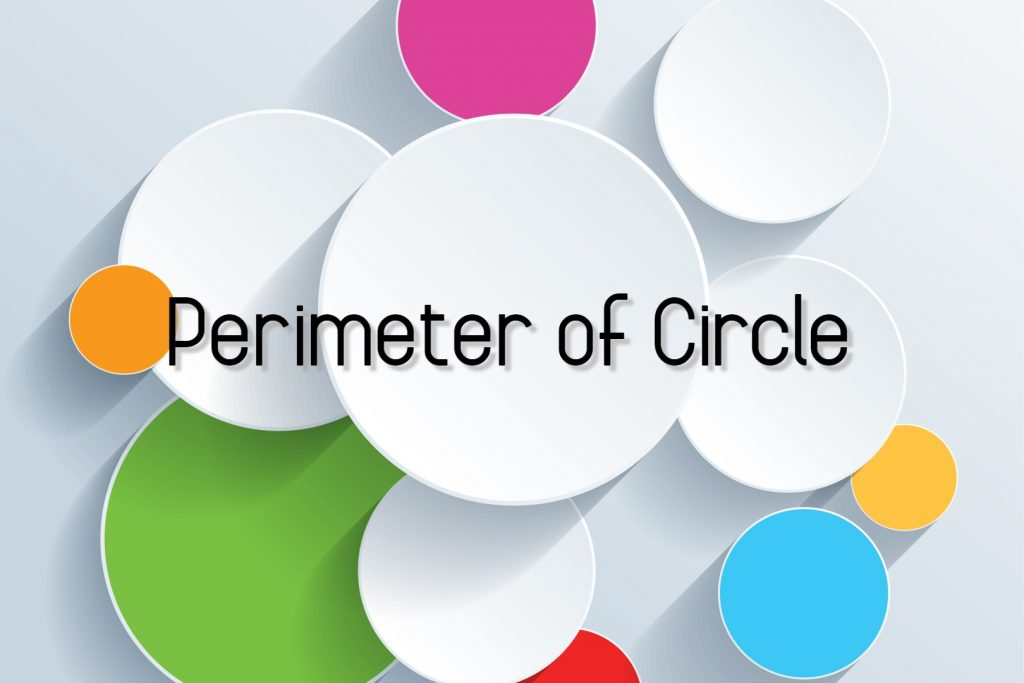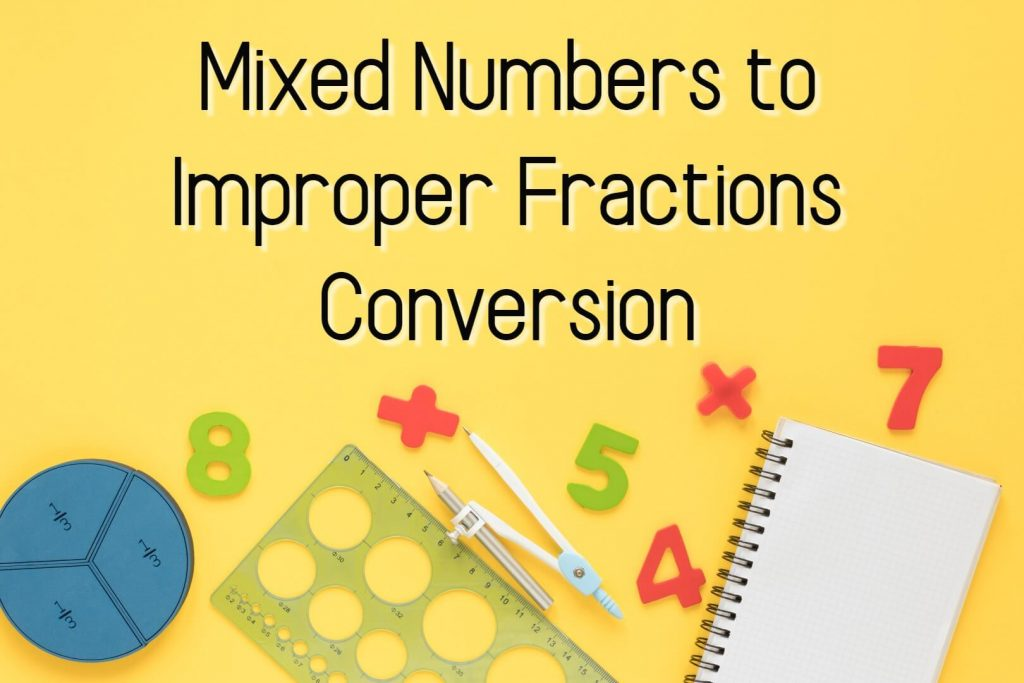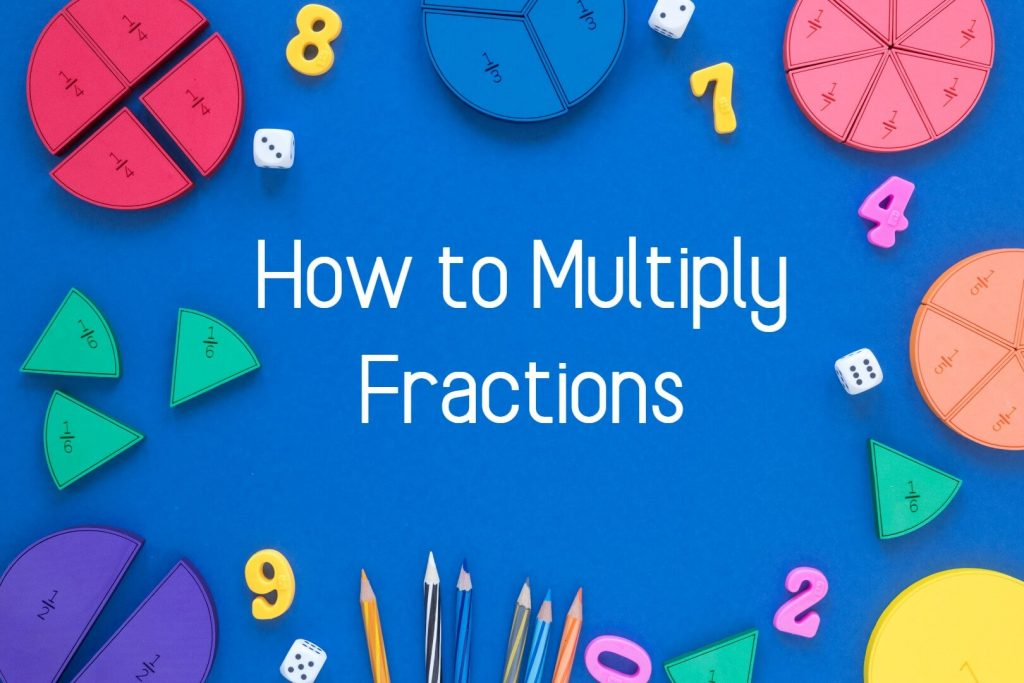## Role of Euclidean Geometry in High-School Mathematics

If only because in one’s “further” studies of mathematics, the results (i.e., theorems) of euclidean geometry appear only infrequently, this subject has come under frequent scrutiny, especially over the past 50 years, and at various stages, its very inclusion during a high-school mathematics curriculum has even been challenged. However, as long as we still think …## Perimeter of Circle

In Perimeter of circle lesson, we are going to discuss, Perimeter of semicircle Perimeter of quarter circle Perimeter of sector What is the Perimeter of Circle Before moving into perimeter let’s recall some knowledge about key features of circle. Center of Circle Diameter of Circle Radius of Circle Center of Circle The center is the …The addition of fractions can discuss in a few categories Unit fraction addition Improper fraction addition Mixed number addition There is only one golden rule that we have to follow when the adding  fractions, There should be a common denominator between the fractions Unit Fraction Addition Example 01: Like Denominators Yellow color area : Green …## Mixed Numbers to Improper Fractions Conversion

In this article, we are going to discuss how to convert Mixed Numbers to Improper Fractions. Before moving into that part, let’s recall what mixed numbers and improper fractions are. A whole pizza and exactly half of an identical pizza shown in the figure. Therefore the total amount of pizza in the picture is 1 …## 3 Steps – How to Multiply Fractions

Multiply fractions can discuss under three main categories. Multiplication of proper fractions  Multiplication of improper fractions Multiplication of a mixed numbers Multiplication of Proper Fractions A proper fraction is a fraction that is less than one, with the numerator less than the denominator. Example : To multiply proper fractions you have to follow only three …